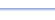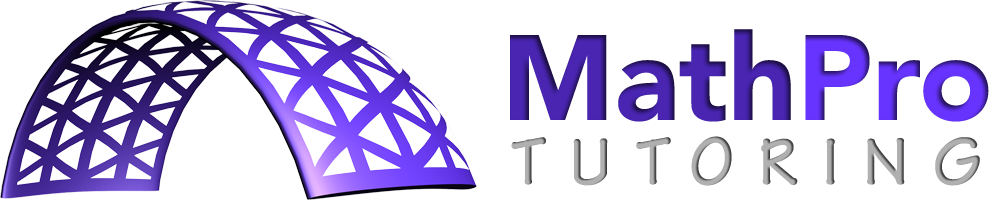Program: MIXEDNUM

MIXEDNUM

• Changes an improper fraction into a mixed number.

Useful for:

• Basic Math
• Algebra 1
• Geometry
• Algebra 2
• Precalculus
• Calculus

See full list of programs

Example

Q: Write 3.4 as a mixed number.

Solution:

First type 3.4 and
press ENTER.

Run MIXEDNUM.  How?

The program reports:

Interpretation:

*Note:  Any rational number can be converted to a mixed number if it has few enough digits for the calculator to display it as a fraction.  If you enter a negative value, the program will report both the whole number and fraction parts as negative.  Just ignore these negatives, write the mixed number, and put a negative sign before it.